Ch 1. Limits Multimedia Engineering Math Limit of aSequence Limit of aFunction LimitLaws Continuity RateChange
 Chapter 1. Limits 2. Derivatives I 3. Derivatives II 4. Mean Value 5. Curve Sketching 6. Integrals 7. Inverse Functions 8. Integration Tech. 9. Integrate App. 10. Parametric Eqs. 11. Polar Coord. 12. Series Appendix Basic Math Units Search eBooks Dynamics Fluids Math Mechanics Statics Thermodynamics Author(s): Hengzhong Wen Chean Chin Ngo Meirong Huang Kurt Gramoll ©Kurt GramollMATHEMATICS - THEORY Slope of a line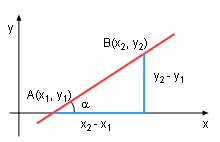Slope of a Line The slope of a line is denoted by m and can be determined with two points: A(x1,y1) and B(x2, y2).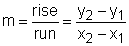It can also be determined by: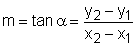in which α is the smallest positive angle from the x axis to the line. When the line is horizontal, m = 0 and when the line is vertical, m is infinity or not defined. Tangent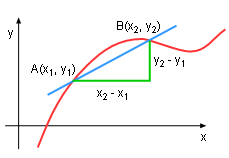Slope of a Curve Suppose curve C (f(x)) is a function of x, the tangent line of the curve C at point A is desired. Now, consider B point where x2 is not equal to x1, the slope of AB is: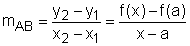Tangent of a Curve Let point B approaches A along the curve C by letting x2 approaches x1, when x2 - x1 is small enough, the slope of AB will overlap with its tangent line at point A.Thus, the tangent at point A can be written as: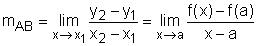Tangent Example - Velocity Velocity is a good example of a tangent line. Normally, the distance s is a function of time t. The velocity of a time interval is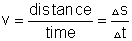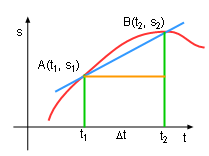Velocity of any Function On a graph of distance plotted as a function of time, the tangent (instantaneous velocity) is: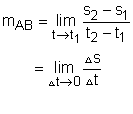Therefore, the instantaneous velocity at time t, is equal to the slope of the tangent line at point A.